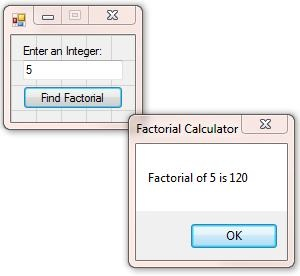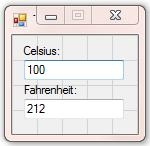#Maths Revision V1.0

This is a suite of 22 revision aid programs + tools I wrote...
You can download the compiled version (msInstaller) here + the zipped source code for the 22 applications here.

(Click the icons to navigate)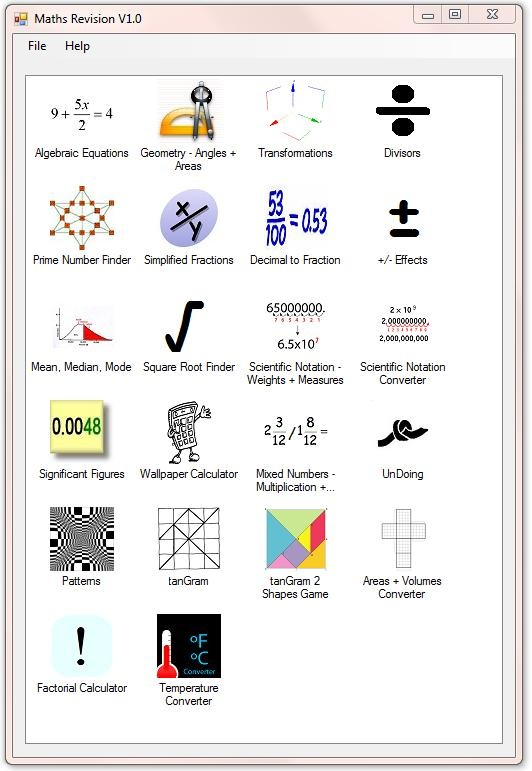My Algebraic Equations program formulates questions for you to answer, + has a scoring system to keep track of your progress: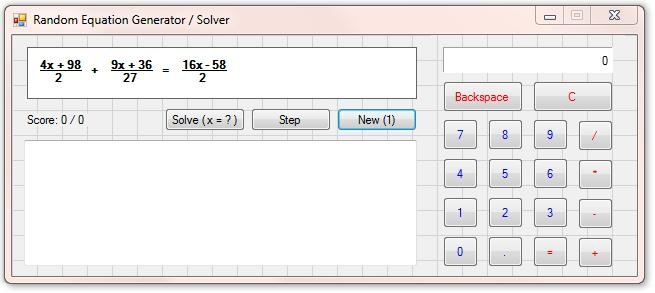My Geometric Shapes + Angles + Areas program is another old one from 2009: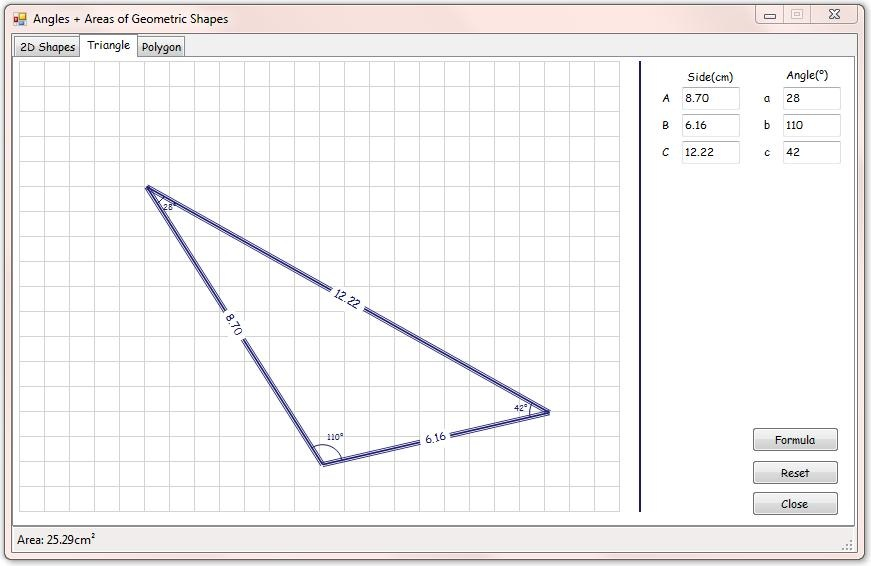This is my Transformations program, written in early 2012. There are 4 different types of transformation: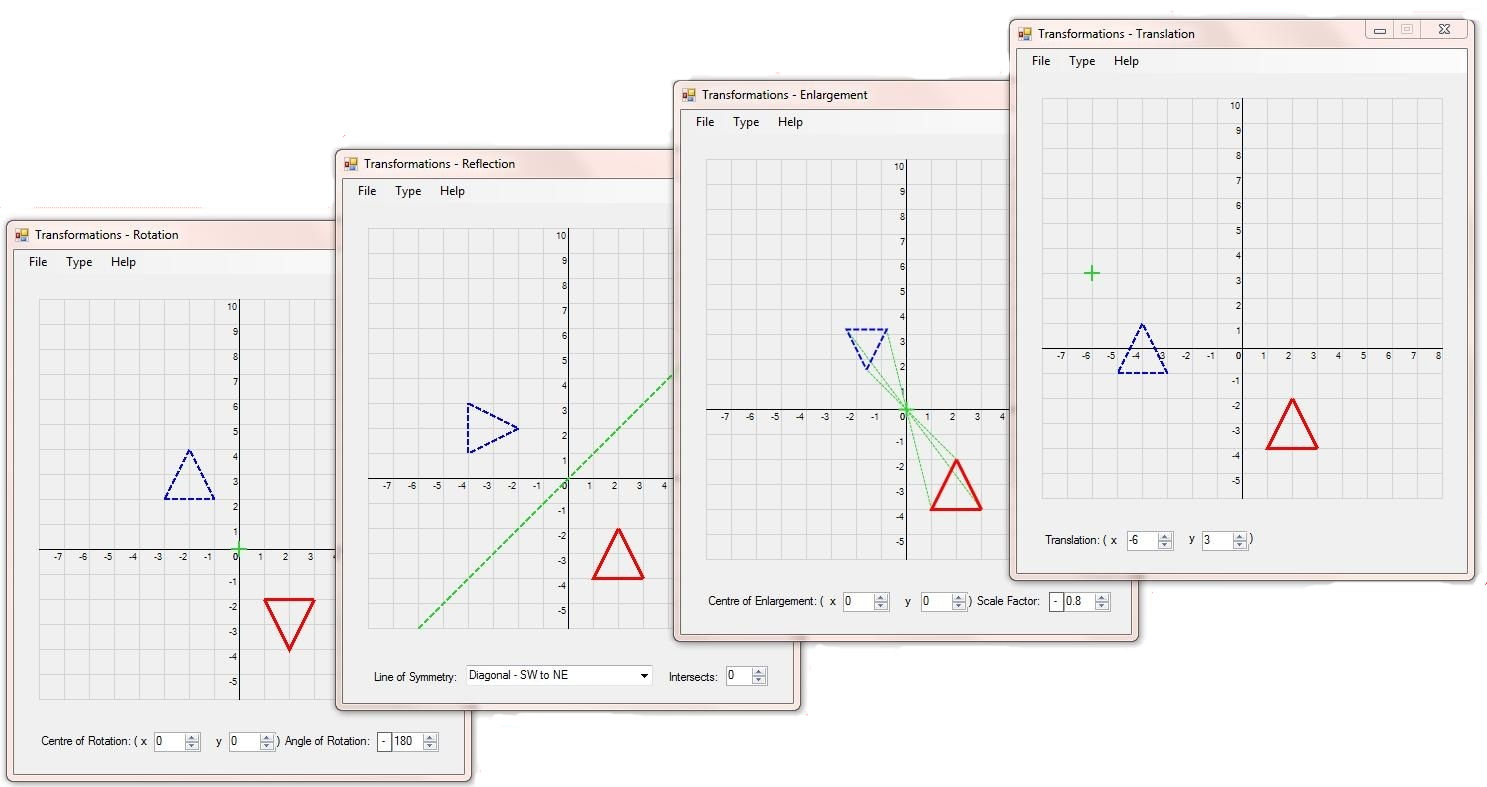This is my Divisors program, written to find all possible divisors of a user entered integer: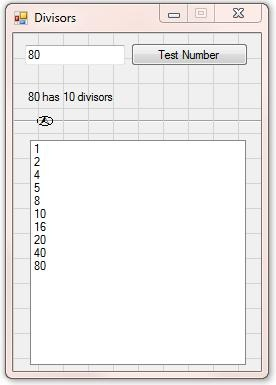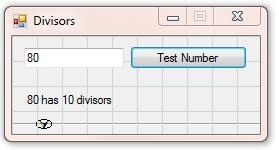Here's my Prime Number Finder. You choose how many prime numbers to find + what number to start at: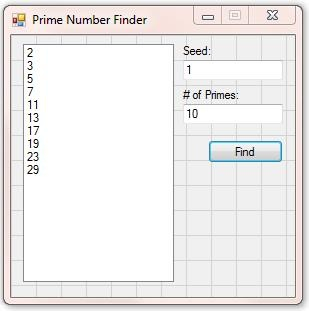This is my Simplified Fractions program + my Decimal to Fraction program: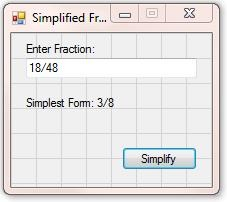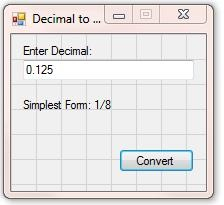This is my -/+ (Positive/Negative) Effects program that demonstrates the effects of performing mathematical operations on positive + negative numbers: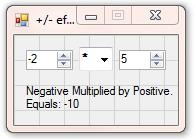This is my Mean, Median, + Mode calculator: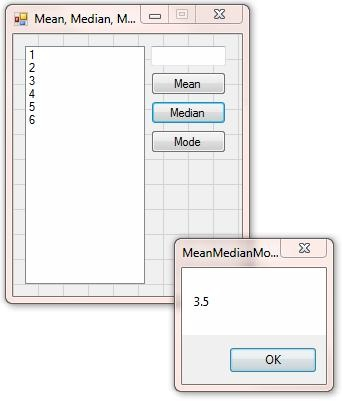This is my Square Root Finder, that uses the pre calculator method of determining the square root of a number: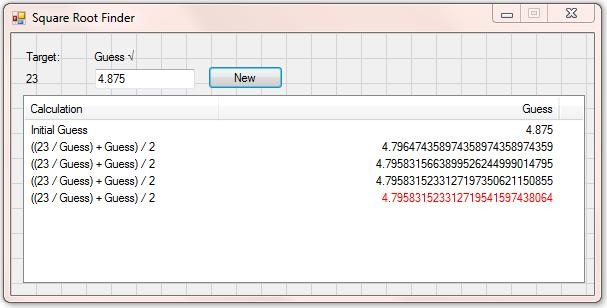This program (top) converts Weights + Measures + outputs in Scientific Notation. The program below converts numbers between decimals + Scientific Notation + vice versa: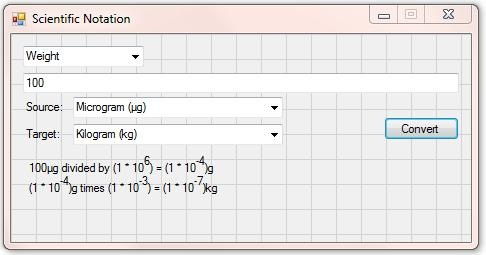This program is a Significant Figures quiz: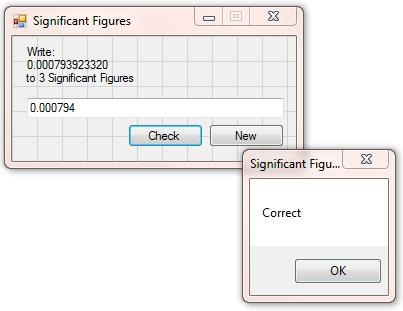This program is a Wallpaper Calculator tool: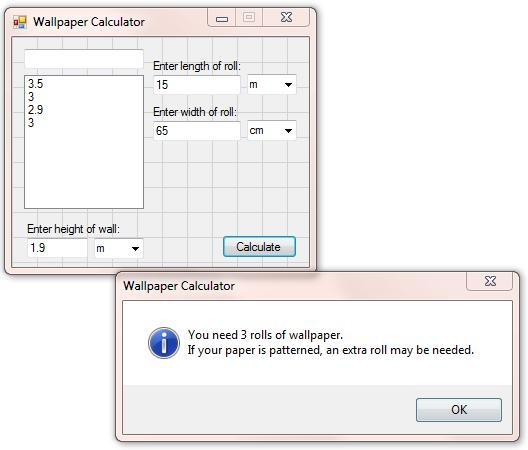This program is a Mixed Numbers Division + Multiplication program, with random formulated questions + a scoring system:This is an UnDoing quiz program, where you must work backwards from the answer to find the value of X: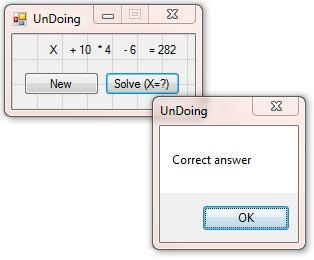This is a Patterns program where you can display various types of pattern + number pattern in a grid comprised of hexagonal cells: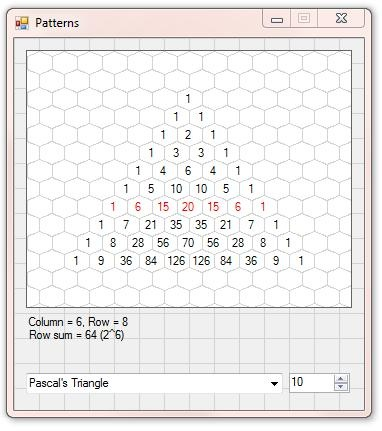These are 2 tanGram programs, first an analytical look at the tanGram, + an interactive tanGram Shapes game: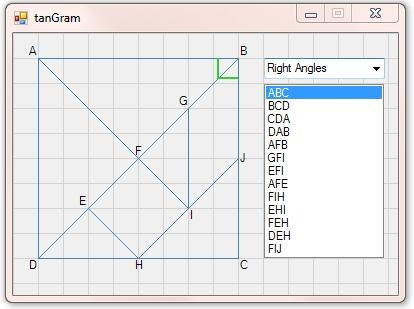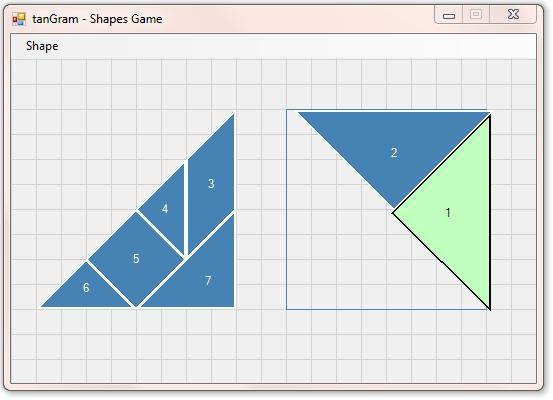This is an Areas + Volumes Converter with graphical conversion solution: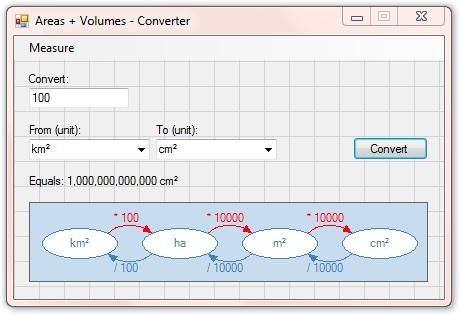This is a Factorial Calculator: